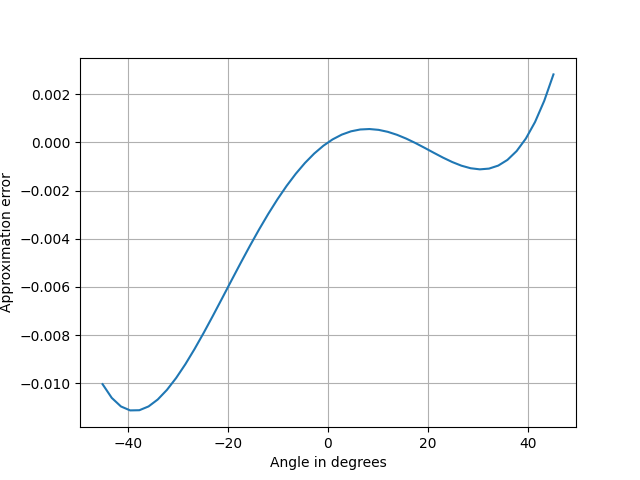# Mentally compute cosine

Ronald Doerfler presents a simple approximation for the cosine of an angle in degrees in his book Dead Reckoning.

cos d° ≈ 1 – d(d + 1)/7000

The approximation is good to about three digits for angles between 0° and 40°.

Although cosine is an even function, the approximation above is not, and the error is larger for negative angles. But there’s no need to use negative values since cos(-d) = cos(d). Just compute the cosine of the absolute value of the angle.The simplest thing to do would have been to use a Taylor approximation, but Taylor approximations don’t work well over a large interval; they’re excessively accurate near the middle and not accurate enough at the ends. The error at 40° would have been 100x larger. Also, the Taylor approach would have required multiplying or dividing by a more mentally demanding number than 7000.

If we wanted to come up with a quadratic approximation for a computer rather than for a human, we could squeeze out more accuracy. The error curve over the interval [0, 40] dips down significantly more than it goes up. An optimal approximation would go up and down about the same amount.